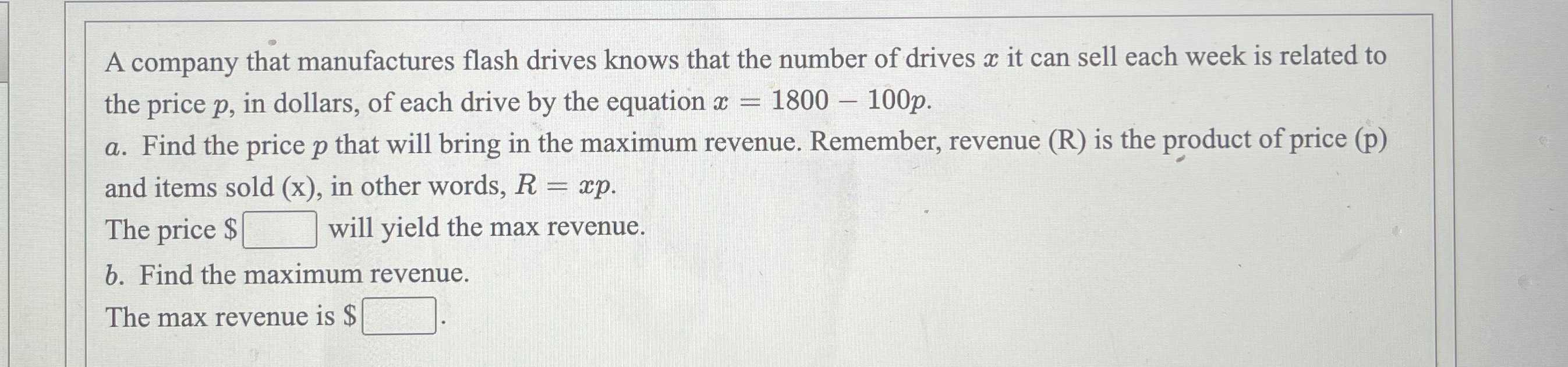### Still have math questions?

Algebra
QuestionA company that manufactures flash drives knows that the number of drives $$x$$ it can sell each week is related to the price $$p$$ , in dollars, of each drive by the equation $$x = 1800 - 100 p$$ . a. Find the price $$p$$ that will bring in the maximum revenue. Remember, revenue $$( R )$$ is the product of price (p) and items sold $$( x )$$ , in other words, $$R = x p$$ .